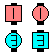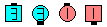Definitions of Square Dance Calls and Concepts

T-Bone \$B\$G\$J\$\$(B 2 x 2 \$B\$+\$i(B.

Trailers \$B\$O(B Facing Recycle \$B\$N<+J,\$N%Q!<%H\$r(B, Leaders \$B\$O(B 1 \$B\$D\$NF0\$-\$H\$7\$F(B Mini-Chase and Hinge \$B\$N<+J,\$N%Q!<%H\$r9T\$\$\$^\$9(B.

\$B1&\$N(B Wave \$B\$G=*\$o\$j\$^\$9(B.Revolve To A Wave\$B\$NA0(B \$B8e(B

\$BCm
• Leader \$B\$N%Q!<%H\$O(B, Rally [C3A] \$B\$N30B&\$N?M\$NF0\$-\$K;w\$F\$\$\$^\$9(B.
• \$B:8\$N(B Mini Wave \$B\$N(B Box \$B\$+\$i\$O(B, Split Recycle \$B\$r\$7\$,\$A\$J\$N\$G(B, \$BCm0U\$,I,MW\$G\$9(B.
• Cheat: Leaders Right-face U-Turn Back; all Facing Recycle.